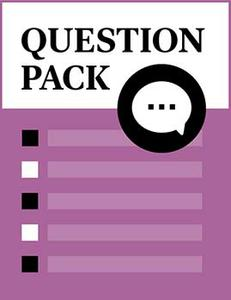# Electricity and Measuring

Source: Skills Commons

Student Price: Free with Top Hat Pro Subscription

A survey of electronics question pack by Pueblo Community College

Atoms and Electricity Q1

All matter is made up of tiny particles called:

A

Ions

B

Molecules

C

Protein

D

None of the above

Atoms and Electricity Q2

Molecules are made up of smaller particles called:

A

Atoms

B

Insulators

C

Proteins

D

None of the above

Atoms and Electricity Q3

Particles found within the atom are:

A

Electrons

B

Protons

C

Neutrons

D

All of the above

E

None of the above

Atoms and Electricity Q4

The ______________ is located in the center of the atom.

A

Proton

B

Electron

C

Nucleus

D

None of the above

Atoms and Electricity Q5

A proton has a ______________ charge.

A

Positive

B

Negative

C

Neutral

Atoms and Electricity Q6
No correct answers: No correct answer has been set for this question

An electron has a ______________ charge.

A

Positive

B

Negative

C

Neutral

Atoms and Electricity Q7

Normally, every atom contains an ______________ number of electrons and protons.

A

Equal

B

Odd

C

Unequal

Sources and Characteristics of Electricity Q8

The first law of electric charges states:

A

Like charges attract

B

Like charges repel

C

Unlike charges attract

D

Unlike charges repel

E

b and c

F

c and d

Sources and Characteristics of Electricity Q9

The three common ways for a neutral object to become charged are:

A

Friction

B

Conduction

C

Induction

D

Current

E

a, b, and c

F

All of the above

Sources and Characteristics of Electricity Q10

A thermocouple :

A

Converts high voltage to low voltage

B

Converts heat energy into electrical energy

C

Converts high current to low current

D

All of the above

E

None of the above

Sources and Characteristics of Electricity Q11

A battery:

A

Converts chemical energy to electrical energy

B

Converts electrical energy to chemical energy

C

Converts current to voltage

D

All of the above

E

None of the above

Sources and Characteristics of Electricity Q12

A piezoelectric device:

A

Converts chemical energy to electrical energy

B

Converts current to voltage

C

Converts mechanical energy to electrical energy

D

All of the above

E

None of the above

Sources and Characteristics of Electricity Q13

A generator:

A

Converts mechanical-magnetic energy to electric energy

B

Converts chemical energy to electrical energy

C

Converts electrical energy to chemical energy

D

Converts current to voltage

E

All of the above

Series and Parallel Circuits Q14

In a series circuit:

A

The current is the same value throughout the circuit

B

The voltage is the same value throughout the circuit

C

The current has different values throughout the circuit

D

The current and voltage are the same value throughout the circuit

Series and Parallel Circuits Q15

In a parallel circuit:

A

The current is the same value throughout the circuit

B

The voltage is the same value throughout the circuit

C

The current has different values throughout the circuit

D

The current and voltage are the same value throughout the circuit

Series and Parallel Circuits Q16

In a series circuit, the voltage across an open component:

A

Is zero volts

B

Is equal to the source voltage

C

Is less than the source voltage

D

Is greater than the source voltage

Series and Parallel Circuits Q17

Two 50 ohm resistors are connected in series to a 12 volt source, what is the voltage drop across each resistor?

A

12 volts

B

10 volts

C

6 volts

D

0 volts

Series and Parallel Circuits Q18

What is the current through each resistor?

A

12 A

B

12 mA

C

120 mA

Series and Parallel Circuits Q19

What is the power dissipated by each resistor?

A

720 mW

B

72 W

C

172 W

Series and Parallel Circuits Q20
No correct answers: No correct answer has been set for this question

What is the total power dissipated in the circuit?

A

720 mW

B

1.44 W

C

1.44 mW

Series and Parallel Circuits Q21

Two 50 ohm resistors are connected in parallel to a 12 volt source, what is the voltage drop across each resistor?

A

12 volts

B

10 volts

C

6 volts

D

0 volts

Series and Parallel Circuits Q22

What is the current through each resistor?

A

24 A

B

240 mA

C

240 A

Series and Parallel Circuits Q23

What is the power dissipated by each resistor?

A

288 W

B

2.88 mW

C

2.88 W

Series and Parallel Circuits Q24

What is the total power dissipated in the circuit?

A

5.76 mW

B

5.76 W

C

576 mW

Measuring Q25

How are multimeters connected in a circuit to measure voltage?

A

Series

B

Parallel

C

Series/parallel

D

None of the above

Measuring Q26

How are multimeters connected in a circuit to measure current?

A

Series

B

Parallel

C

Series/parallel

D

None of the above

Measuring Q27

What device is used primarily to measure current in the field?

A

Voltage probe

B

Power probe

C

Current probe

Measuring Q28

What does this device measure specifically?

A

The strength of the magnetic field generated by the conductor

B

The strength of the voltage generated by the conductor

C

The power of the conductor

Measuring Q29

How is resistance measured?

A

In parallel with power on

B

In series with power off

C

With the power off and one end of the component disconnected from the circuit